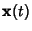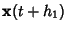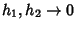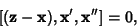## Osculating Plane

The Plane spanned by the three points,, andon a curve as. Letbe a point on the osculating plane, thenwheredenotes the Scalar Triple Product. The osculating plane passes through the tangent. The intersection of the osculating plane with the Normal Plane is known as the Principal Normal Vector. The Vectorsand(Tangent Vector and Normal Vector) span the osculating plane.